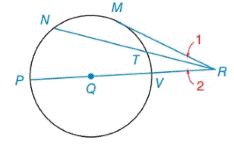Chapter 6.2, Problem 7E### Elementary Geometry for College St...

6th Edition
Daniel C. Alexander + 1 other
ISBN: 9781285195698

#### Solutions

Chapter
Section### Elementary Geometry for College St...

6th Edition
Daniel C. Alexander + 1 other
ISBN: 9781285195698
Textbook Problem
2 views

# Given: In ⊙ Q , P R ¯ contains Q, M R ¯ is a tangent, m M P ⌢ = 112 ° , m M N ⌢ = 60 ° and m M T ⌢ = 46 ° Find: a) m ∠ M R P b) m ∠ 1 c) m ∠ 2To determine

(a)

To find:

mMRP when Q, PR¯ contains Q, MR¯ is a tangent, mMP=112°, mMN=60° and mMT=46°.

Explanation

Theorem:

The measure of an angle formed by a tangent and a secant that intersect at a point outside the circle is one-half the difference of the measures of the two intercepted arc.

Calculation:

mMRP when Q, PR¯ contains Q, MR¯ is a tangent, mMP=112°, mMN=60° and mMT=46°

mMP+mMT+mTV=180°112°+46°+mTV=180°158°+mTV=180°m

To determine

(b)

To find:

m1 when Q, PR¯ contains Q, MR¯ is a tangent, mMP=112°, mMN=60° and mMT=46°.

To determine

(c)

To find:

m2 when Q, PR¯ contains Q, MR¯ is a tangent, mMP=112°, mMN=60° and mMT=46°.

### Still sussing out bartleby?

Check out a sample textbook solution.

See a sample solution

#### The Solution to Your Study Problems

Bartleby provides explanations to thousands of textbook problems written by our experts, many with advanced degrees!

Get Started

#### What are the two requirements for a random sample?

Statistics for The Behavioral Sciences (MindTap Course List)

#### If sin=47, find csc.

Trigonometry (MindTap Course List)

#### In Exercises 2532, find the inverse of the matrix if it exist. A=

Finite Mathematics for the Managerial, Life, and Social Sciences